# TI-84 Plus CE online calculator applications

Built-in calculator apps extend the capabilities of the TI-84 Plus CE online calculator. These apps, also available on the TI-84 Plus CE graphing calculator, allow you to explore conics, inequalities, polynomials and more.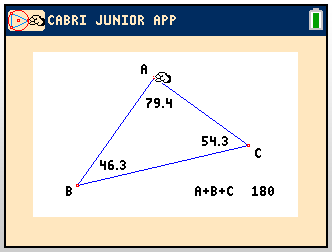## Cabri™ Jr. App

Now you can add a new dimension to your students’ learning experience with interactive geometry for your calculator. Create excitement in the classroom as you construct, analyze, and transform mathematical models and geometric diagrams.

The Cabri™ Jr. App was developed for TI by CABRILOG® with renowned French mathematician Jean-Marie Laborde. Just take a look at what the Cabri™ Jr. App can do:

• Perform analytic, transformational and Euclidean geometric functions
• Build geometric constructions interactively with points, a set of points for locus, lines, polygons, circles, and other basic geometric objects
• Alter geometric objects on the fly to see patterns, make conjectures, and draw conclusions — plus, you’ll get a more intuitive and highly interactive interface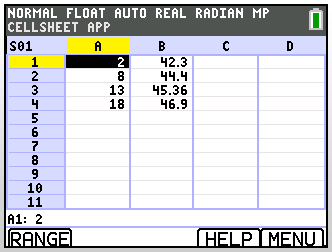## CellSheet™ App

Your calculator can become a more versatile tool to use in a variety of classes with the addition of spreadsheet capabilities:

• Allows the student to enter data and text in cells
• Create cell formulas and use built-in functions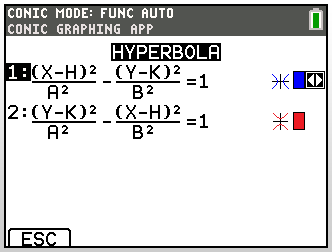## Conic Graphing App

This app will present equations in function, parametric or polar form and provides a simple way to graph the four conic shapes:

• Circle
• Ellipse
• Hyperbola
• Parabola

Enter the required parameters to:

• Graph
• Trace
• Solve for the conic’s characteristic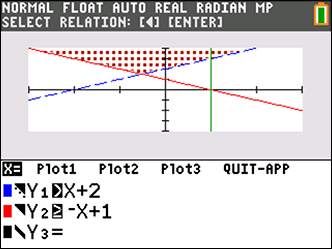## Inequality Graphing App

Students can enter inequalities using symbols, plot inequalities including union and intersection shades, trace points of interest (intersections) between functions, store (x,y) coordinate pairs for viewing and enter Linear Programming functions.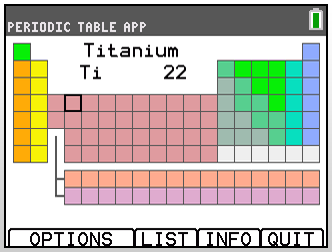## Periodic Table App

This app is not just your basic periodic table — students can also study and review trends in the periodic table easily with their TI graphing calculator.

• Fifteen properties and facts about the known elements
• Graphs of the periodic nature of the elements
• Easy navigation between the elements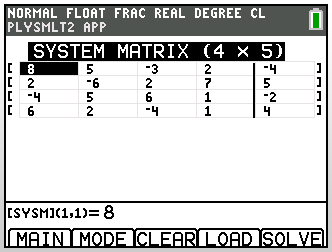## Polynomial Root Finder and Simultaneous Equation Solver App

Polynomial Root Finder App capabilities include:

• Enter polynomials up to and including order (degree) 10
• Easy to use POLY MODE screen to set up all options
• Display roots as fractions or decimals for many roots
• Choose to display only Real roots for 2nd and 3rd degree polynomials
• For polynomials of degree 4 and higher, roots display in Complex format
• Store polynomials to Y= for graphing and evaluation
• Verify a root is the zero of the polynomial function by storing roots in Real format

Simultaneous Equation Solver App capabilities include:

• Enter systems of equations with up to 10 equations and 10 unknowns
• Easy to use SIMULT MODE screen to set up all options
• Displays unique solution, infinite solution and no solution
• Store coefficient matrix, augmented matrix and the solution
• Displays Reduced Row Echelon form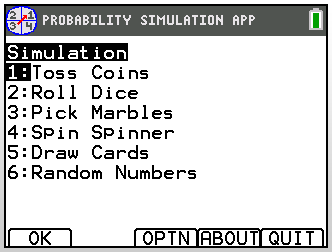## Probability Simulation App

Explore probability theory with interactive animation that simulates the rolling of dice, tossing of coins and generating random numbers on your calculator.

Options include:

• Bar Graph
• Table of trials data
• Settings for specifying numbers of trials
• Ways to collect data
• Weighting

In addition, students can export data for further exploration.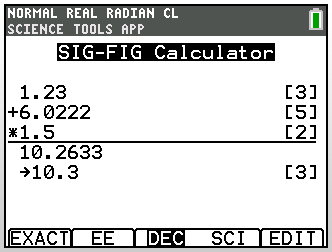## Science Tools App

The Science Tools App provides the capability to perform unit conversions on your calculator. Get more use from your calculator in the science classroom with this app.

App elements include:

• Constants and conversions
• Significant figures calculator
• Graphing tool
• Vector tool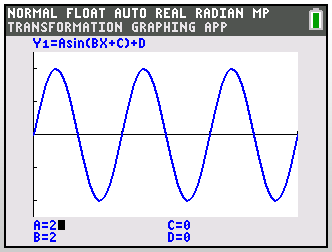## Transformation Graphing App

Students can visually draw conclusions about functions and improve graphing comprehension with this app. To take advantage of this app’s capabilities, simply input functions, and view changes in the function as the parameters are changed.

Students can:

• Instantly see how changing the value of a coefficient transforms a graph
• Visually fit equations to data plots by manipulating coefficients

## Language localization apps

These apps will translate all prompts, error messages and most functions into French, German, Spanish, Dutch, Swedish and Portuguese.

CABRILOG is a registered trademark of CABRILOG SAS.